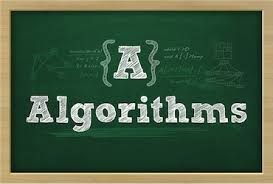11. How to find median of a BST?

Find the no. of elements on the left side.
If it is n-1 the root is the median.
If it is more than n-1, then it has already been found in the left subtree.
Else it should be in the right subtree
12. What is Diffie-Hellman?

It is a method by which a key can be securely shared by two users without any actual exchange.

13. What is the goal of the shortest distance algorithm?
The goal is completely fill the distance array so that for each vertex v, the value of distance[v] is the weight of the shortest path from start to v.

14. Explain the depth of recursion?

This is another recursion procedure which is the number of times the procedure is called recursively in the process of enlarging a given argument or arguments. Usually this quantity is not obvious except in the case of extremely simple recursive functions, such as FACTORIAL (N), for which the depth is N.

15. Explain about the algorithm ORD_WORDS?

This algorithm constructs the vectors TITLE, KEYWORD and T_INDEX.

16. Which are the sorting algorithms categories?

Sorting algorithms can be divided into five categories:
a) insertion sorts
b) exchange sorts
c) selection sorts
d) merge sorts
e) distribution sorts

17.Define a brute-force algorithm. Give a short example.

A brute force algorithm is a type of algorithm that proceeds in a simple and obvious way, but requires a huge number of steps to complete. As an example, if you want to find out the factors of a given number N, using this sort of algorithm will require to get one by one all the possible number combinations.

18. What is a greedy algorithm? Give examples of problems solved using greedy algorithms.

A greedy algorithm is any algorithm that makes the local optimal choice at each stage with the hope of finding the global optimum. A classical problem which can be solved using a greedy strategy is the traveling salesman problem. Another problems that can be solved using greedy algorithms are the graph coloring problem and all the NP-complete problems.

19. What is a backtracking algorithm? Provide several examples.

It is an algorithm that considers systematically all possible outcomes for each decision. Examples of backtracking algorithms are the eight queens problem or generating permutations of a given sequence.

20. What is the difference between a backtracking algorithm and a brute-force one?

Due to the fact that a backtracking algorithm takes all the possible outcomes for a decision, it is similar from this point of view with the brute force algorithm. The difference consists in the fact that sometimes a backtracking algorithm can detect that an exhaustive search is unnecessary and, therefore, it can perform much better.